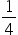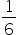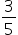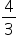science
Easy

Question

# A car moving at a velocity of 5 m/s accelerates to 25 m/s while covering a distance of 1.8 km. The acceleration of the car is ___ m/s2.

##Hint:

## The correct answer is:### Given:Initial velocity (u) = 5ms-1Final velocity (v) = 25ms-1Distance (s) = 1.8Km or 1800sTo find:Acceleration (a) = ?Solution:Answer:Acceleration of the car is 1/6ms-2#### With Turito Foundation.#### Get an Expert Advice From Turito.